Lemniscate of Gerono
LEMNISCATE OF GERONO

Balmoral Software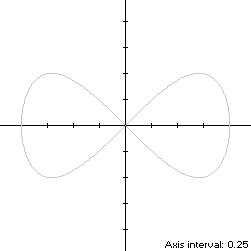The Lemniscate of Gerono is a bisymmetric figure-eight curve S with parametric equations
x(t) = sin(t)

y(t) = sin(2t)/2, 0 ≤ t < 2π

The path of S starts at the origin, passes clockwise through Quadrants I and IV, then counterclockwise through Quadrants II and III and back to the origin. Its abscissa extrema are on the x-axis at ±1 when t = ±π/2, and its extreme ordinate points are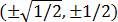at t = ±π/4, ±3π/4, so the width x height of its bounding rectangle is 2 x 1.

### Metrics

The perimeter of S is 6.097223 (OEIS A118178) and its area is 4/3.

### Convex Hull

The convex hull is created by connecting the extreme ordinate points with two horizontal line segments of length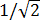. We have
x'(t) = cos(t)

y'(t) = cos(2t),

so by (L1), the perimeter of the convex hull is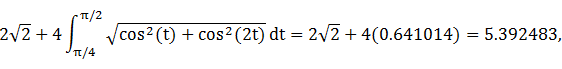which is about 12% shorter than that of the lemniscate.

The line segments of the convex hull create a rectangle of area, as shown in blue in the left diagram below. By (A1), the area of the convex hull is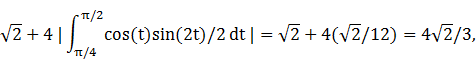which is about 41% more than the area of the lemniscate.

### Circumcircle

The maximum squared distance
x2(t) + y2(t) = sin2(t) + sin2(2t)/4
is 1, so that is the circumradius.

### Circumellipse

From Lemma B, we have
x(t)y(t) = sin(t)sin(2t)/2
This expression is maximized in the first quadrant at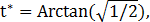so the circumellipse dimensions are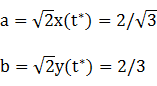For verification, we have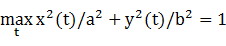### Incircle (lobe)

Consider the right lobe of the lemniscate, where 0 ≤ t < π. The maximum ordinate 1/2 of the lobe does not define its inradius since the corresponding abscissais too close to the right edge (1,0), so its incircle is constrained by the right edge of S. Using z = 1 in Lemma C, we have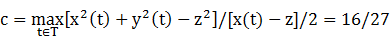and R = |c - z| = 11/27. For verification, we have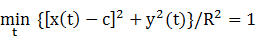### Inellipse (lobe)

Using z = 1 in Lemma E,
d/dt [x(t) - z]y(t) = [sin(t) - 1]sin(2t)/2
has a zero at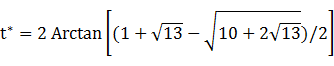The corresponding coordinates are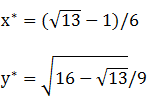We then have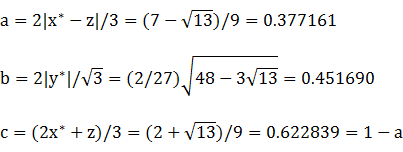For verification, we have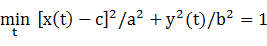### Summary Table

 Perimeter Area Centroid Figure Parameters Incircle (lobe) R = 11/27 2.559816 0.521444 (0.592593,0) Inellipse (lobe)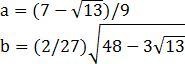2.609178 0.535201 (0.622839,0) Lemniscate of Gerono Width: 2Height: 1 6.097223 1.333333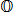Convex hull 5.392483 1.885618 Circumellipse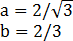5.825171 2.418401 Circumcircle R = 1 6.283185 3.141593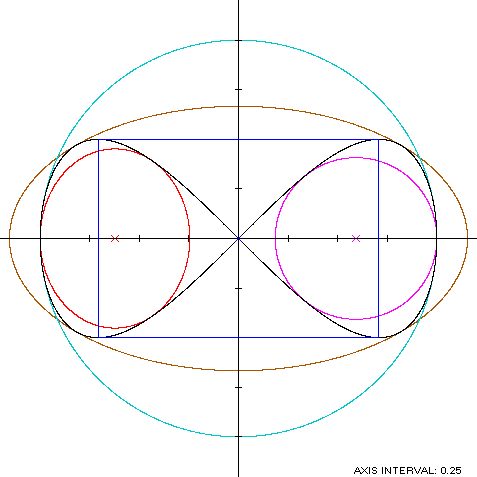Binoculars

The Lemniscate of Gerono (red) is a member of a group of figure-8 curves described on these pages, including (inside to outside) the dumbbell curve, the bowtie, the Lemniscate of Bernoulli and the dipole: